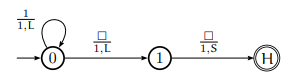# Construct a Turing machine for adding 2 to the binary natural number?

A Turing machine (TM) can be formally described as seven tuples −

(Q,X, ∑, δ,q0,B,F)

Where,

• Q is a finite set of states.

• X is the tape alphabet.

• ∑ is the input alphabet.

• δ is a transition function:δ:QxX->QxXx{left shift, right shift}.

• q0 is the initial state.

• B is the blank symbol.

• F is the final state.

Input − n a natural number

Output − n + 2

Let’s represent natural numbers in unary form (e.g. 3 = 111, 5 = 11111) and 0 will be represented by the empty symbol.

Algorithm

Move the tape head to the left of the first 1 (if it exists).

Change that empty cell to a 1.

Move left and repeat.

Halt

We only need 3 states: 0 (initial), 1 and Halt; as well as three instructions −

(1): (0, 1, 1, L, 0) Move left to blank cell.

(2): (0, , 1, L, 1) Write 1 into the cell and move left.

(3): (1, ❑, 1, S, Halt) Write 1 into cell and halt.

Graphically, it is represented as follows:Instantaneous description

State 0: ❑ 1 1 1 ❑ Begin in state 0

State 0: ❑ 1 1 1 ❑ (1)

State 1: ❑ 1 1 1 1 ❑ (2)

Halt: ❑ 1 1 1 1 1 ❑ (3)